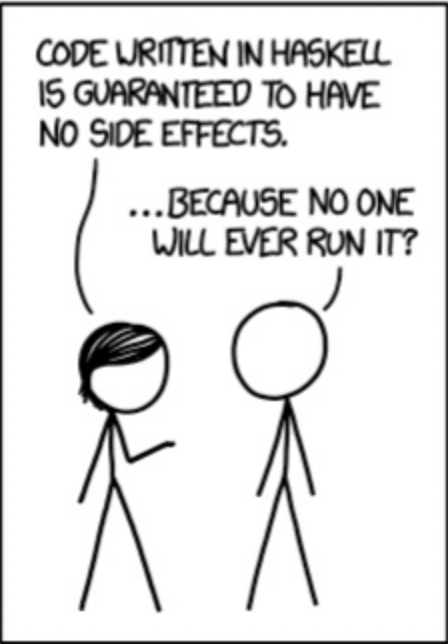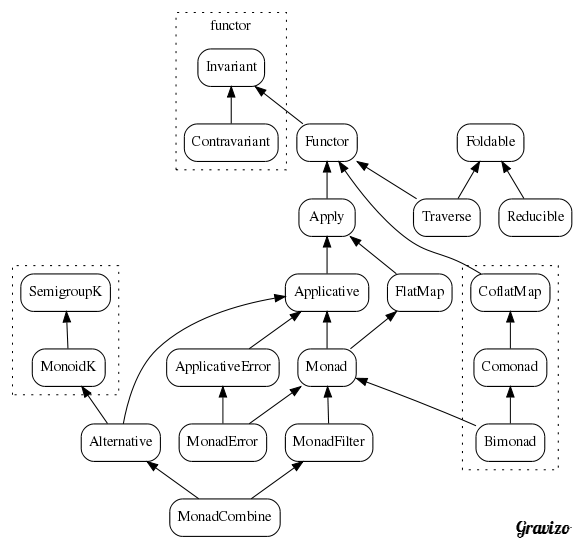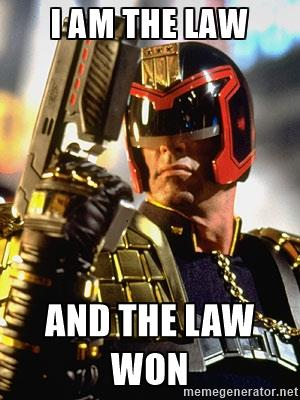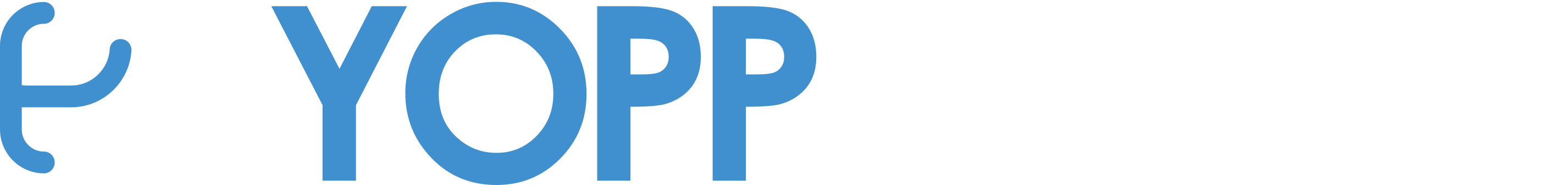## Expressions Oriented

``````42
x = 41 + 1
y = x * 2
``````x = 42
y = x + 1 == 42 + 1``````
``````x = (2 * 2) + (3 * 4)
x = 2 * 2 + 3 * 4
x = 4 + 12
x = 16``````
``````
val add1: Int => Int = (i:Int) => i + 1
val sub1: Int => Int = (i:Int) => i - 2

def compose[A, B, C](
f: B => C,
g: A => B,
): A => C = { a => f(g(a)) }

``````
``````
(Int, Int) => Int becomes Int => Int => Int``````
``````
val sum:     (Int, Int) => Int = (x, y) => x + y
val curried: Int => Int => Int = x => (y => x + y)

curried(1)(3) == 4
``````

"Currying is the technique of translating the evaluation of a function that takes multiple arguments into evaluating a sequence of functions, each with a single argument." - Wikipedia
``````
val f3: (Int, Int, Int) => Int = (x, y, z) => x + y + z
val f2: (Int, Int) => Int = f3(0, _, _)

f2(40, 2) == 42
``````

"Partial application refers to the process of fixing a number of arguments to a function, producing another function of smaller arity" - Wikipedia
• Total: A function must yield a value for every possible input.
• Pure: A function’s only effect must be the computation of its return value
• Deterministic: A function must yield the same value for the same input.

Taken from "A Beginner-Friendly Tour through Functional Programming in Scala".
John A De Goes.

## Inputs / Outputs

``````
def foo(number: String): Int = number.toInt

def bar(input: String): Int = {
println("performing bar")
foo(input)
}``````

## Side Effects

``````
def parse(integer: String): Int = {
if(numberIsNotAString(number)) {
throw new IllegalArgumentException(/*...*/)
} else {
// parsing logic returning parsed integer
}
}``````
``````
def ask(question: String): String = {
if(question == "What's the meaning of life?") {
"42"
else
null // does not compile in Scala
}``````
``````
var n = 41
def add1(y: Int): Int = {
n = n + 1
y + 1
}
// ...
assert(n == 42)``````
``````
def readIntegerFromFile(filePath: String): Int = {
// throws exceptions
// ...
}``````
"A function having a side-effect is a function which has an observable interaction with its calling functions or the outside world besides returning a value"
Wikipedia.
``````
// List.scala
override def drop(n: Int): List[A] = {
var these = this
var count = n
while (!these.isEmpty && count > 0) {
these = these.tail
count -= 1
}
these
}``````## Total Functions

Total: A function must yield a value for every possible input.

``````
def parse(integer: String): Int = {
if(numberIsNotAString(number)) {
throw new IllegalArgumentException(/*...*/)
} else { /* parsing logic */}
}``````
``````
def ask(question: String): String = {
if(question == "What's the meaning of life?") {
"42"
else
null // does not compile in Scala
}``````

``````
trait Either[A, B]
case class Left[A, B](a: A)  extends Either[A, B]
case class Right[A, B](b: B) extends Either[A, B]

def parse(integer: String): Either[Exception, Int]``````
``````
trait Maybe[A]
case class Some[A](a: A) extends Maybe[A]
case class None[A]()     extends Maybe[A]

``````

``````
case class Foo(first: String, second: Int)
``````
``````
Foo("bar", 42) match {
case Foo(x, 42)    => println(s"\$x == 42")
case Foo("bar", _) => println(s"bar != 42")
case _             => println("Default case")
}
``````
``````
Foo("bar", 74) match {
case Foo(x, 42)    => println(s"\$x == 42")
case Foo("bar", _) => println(s"bar != 42")
case _             => println("Default case")
}
``````

``````
def ask(question: String): Maybe[String] = {
if(question == "What's the meaning of life?") {
Some("42")
else
None
}

ask("What's the meaning of life?") match {
case None          =>
}
``````

## Pure Functions

Pure: A function’s only effect must be the computation of its return value

``````
var n = 41
def add1(x: Int): Int = {
n = n + 1
x + 1
}
// ...
assert(n == 42)``````

``````
def add1(x: Int, y: Int): (Int, Int) = {
(x + 1, n + 1)
}
// ...
val n = 41
val (m, n2) = add1(41, n)
assert(n2 == 42)``````

## Deterministic Functions

Deterministic: A function must yield the same output for the same input.

``````
printOnConsole(s)
``````

``````
trait ConsoleIO
case class Read(process: String => ConsoleIO) extends ConsoleIO
case class Write(line: String, then: ConsoleIO) extends ConsoleIO
case object End extends ConsoleIO
``````
``````
val program: ConsoleIO = Read { line => Write(line, End) }
``````

``````
def interpret(program: ConsoleIO): Unit = program match {
case Write(line, next) => println(line); interpret(next)
case End => println("Done !")
}
``````

``````
trait ConsoleIO
case class Read(process: String => ConsoleIO) extends ConsoleIO
case class Write(line: String, then: ConsoleIO) extends ConsoleIO
case object End extends ConsoleIO

val program: ConsoleIO = Read { line => Write(line) }
interpret(program)
``````
``````
def interpret(program: ConsoleIO): Unit = program match {
case Write(line, next) => println(line); interpret(next)
case End => println("Done !")
}
``````
• Total: A function must yield a value for every possible input.
• Pure: A function only effect must be the computation of its return value
• Deterministic: A function must yield the same value for the same input.
•
• Side effects break Referential Transparency :(
• Side-Effects must be explicit
• Separate the 'what' from the 'how'

## Composition

``````
trait Either[A, B]
case class Left[A, B](a: A)  extends Either[A, B]
case class Right[A, B](b: B) extends Either[A, B]
``````
``````
val foo: Either[Exception, Int] = ???
val result = foo match {
case Right(y) => y + 1
case Left(ex) => ??? // what are we supposed to return here???
}
println(result)
``````
``````
val success: Either[Exception, Int] = Right(42)

val failure: Either[Exception, Int] =
Left(new RuntimeException())

map( _ + 1 )(success) // returns 43
map( _ + 1 )(failure) // return failure

def map[A, B](f: A => B)
(e: Either[Exception, A]): Either[Exception, B] =
e match {
case Right(v) => Right(f(v))
case Left(e) => Left(e)
}
``````
``````
val foo: Either[Exception, Int] = ???
val bar: Int => Either[Exception, String] = ???

// meh !
val fooBar: Either[Exception, Either[Exception, String]] =
map(bar)(foo)

val fooBar: Either[Exception, String] = chain(foo, bar)
``````
``````
def chain[E, B, C](f: B => Either[E, C])
(e: Either[E, B]): Either[E, C] = e match {
case Right(b) => f(b)
case Left(e) => Left(e)
}
``````
``````
def map[A, B](f: A => B)(m: Maybe[A]): Maybe[B] =
m match {
case Some(a) => Some(f(a))
case None() => None()
}

def chain[A, B](f: A => Maybe[B])(m: Maybe[A]): Maybe[B] =
m match {
case Some(a) => f(a)
case None() => None()
}
``````
``````
// Algebraic data types
1, 2, 3...

// Operations
1 + 2 = 3
3 * 2 = 6

// Laws
0 + x = x
1 * x = x
``````
``````
def map[A, B](f: A => B)(m: Maybe[A]): Maybe[B] = m match {
case Some(a) => Some(f(a))
case None() => None()
}
``````
``````
def noop[A](a: A): A = a
map(noop)(foo) == foo
``````
``````
val f: Int => Int = ???
val g: Int => String = ???

map(compose(f, g))(foo) == compose(map(f),map(g))(foo)
``````
``````
trait Functor[F[_]] {
def pure[A](a: A): F[A]
def map[A, B](fa: F[A], f: A => B): F[A]
}               ``````
``````
# identity law
map(noop)(foo) == foo

# composition law
map(compose(f, g))(foo) == compose(map(f),map(g))(foo)
``````
``````
val foo: Int => Int => String = x => y => s"\$x, \$y"

val m1: Maybe[Int] = Some(1)
val m2: Maybe[Int] = Some(2)
``````
``````
val pFoo: Maybe[Int => String] =
map(foo)(m1) // Some(y => "1, \$y")
``````
``````
def ap[A, B](mf: Maybe[A => B])(ma: Maybe[A]): Maybe[B] =
ma match {
case Some(a) => map(f => f(a))(mf)
case None()  => None()
}
``````
``````
val result: Maybe[String] = ap(pFoo)(m2)
``````
``````
trait Applicative[F[_]] extends Functor[F] {
def ap[A, B](ff: F[A => B])(fa: F[A]): F[B]
}
``````
``````
ap(ff)(fx) == map(f)(fx)
``````
``````
def chain[A, B](f: A => Maybe[B])(m: Maybe[A]): Maybe[B] =
m match {
case Some(a) => f(a)
case None() => None()
}
``````
``````
val f: Int => Maybe[Int] = ???
val pure: Int => Maybe[Int] = (i: Int) => Some(i)
``````
``````
chain(pure)(Some(42)) == Some(42)
``````
``````
chain(f)(Some(42)) == f(42)
``````
``````
val g: Int => Maybe[Int] = ???

chain(chain(Some(42), f), g)
== chain(Some(42), i => chain(f(i), g))
``````
``````
def flatMap[A, B](ma: M[A], f: A => M[B]): M[A]
}               ``````
``````
// Left identity law
chain(pure)(Some(42)) == Some(42)

// Right identity law
chain(f)(Some(42)) == f(42)

// Composition law
chain(chain(Some(42), f), g)
== chain(Some(42), i => chain(f(i), g))
``````
``````
trait Functor[F[_]] {
def pure[A](a: A): F[A]
def map[A, B](fa: F[A], f: A => B): F[A]
}

def flatMap[A, B](ma: M[A], f: A => M[B]): M[A]
}

trait Applicative[F[_]] extends Functor[F] {
def lift[A, B](ff: F[A => B])(fa: F[A]): F[B]
}
``````## Conclusion

• Learn You a Haskell for Great Good! : by Miran Lipovaca
• Functional Programming in Scala : by Paul Chiusano and Rúnar Bjarnason
• http://degoes.net/: by John A De GoesThank you ! Questions ?